# Module 5 - Time Series Modeling

In module four (4), we demonstrated correlogram analysis and its use in identifying proper time series models.

In this module, we will walk you through the model specification process using NumXL functions and tools.

NumXL supports numerous time series models: ARMA, ARIMA, AirLine, GARCH, etc., and more will be added as users request them.

In all cases, we start this phase with a model in mind (e.g. GARCH(1,1)) and use NumXL tools and wizards to facilitate the model specification stage.

For the sample data, we are using the weekly log returns for the S&P 500 between January 2009 and July 2012.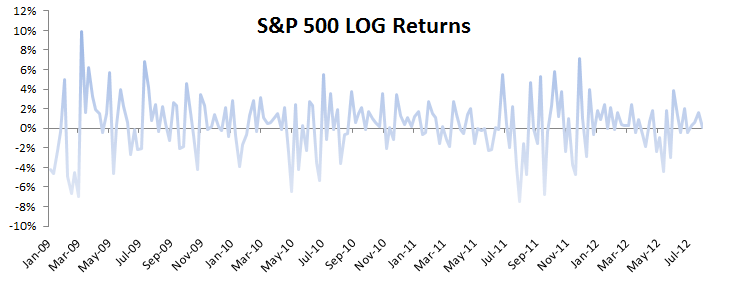In Module 4, we showed that the weekly log returns don’t exhibit significant serial correlation, but they do possess an ARCH effect. In other words, an ARCH/GARCH model is more suited to fit the data than, say, ARMA. For start, let’s consider a GARCH(1,1) model:

$$y_t=\mu + a_t\\a_t=\sigma_t \times \epsilon_t\\\epsilon_t \sim \Phi(0,1) \\\sigma_t^2 = \alpha_o + \alpha_1 a_{t-1}^2 + \beta_1 \sigma_{t-1}^2$$

Using NumXL toolbar, locate and click on the GARCH icon.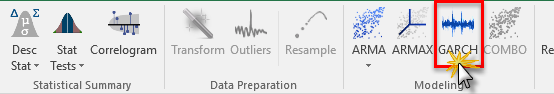The GARCH wizard dialog box pops up. In the input data field, specify the cells range for the sample data. Next, enter the values of the ARCH and GARCH component orders as one (1).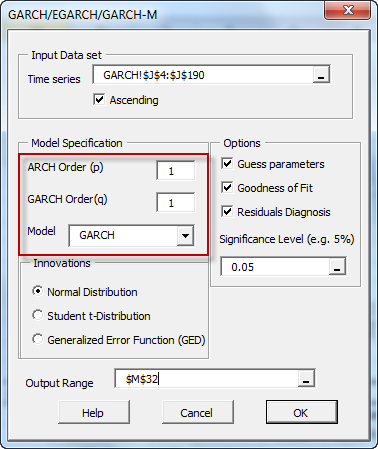For innovations distribution, we’ll use the default – Gaussian distribution, and this completes the GARCH(1,1) model specification.

Next, let’s instruct the GARCH wizard to generate and augment the goodness-of-fit calculations and residuals diagnosis sections in the model output table.

By default, the selected cell is used for the output range value. If this is acceptable, let’s click the OK button.

The following table will be generated in your worksheet: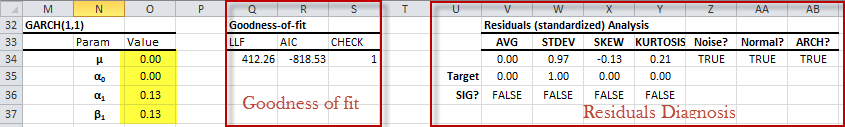The model’s parameter values are set by a quick guess, and they are not optimal. The model ought to be calibrated (next module) before we can gauge its fit or consider it for forecasting.

In the middle table (i.e. Goodness of fit), the wizard created a log-likelihood function and Akaike information criterion formulas in the corresponding cells. The formulas reference the model’s parameters cells and input data range, so after you calibrate the model, they will reflect the goodness of fit of the optimal values.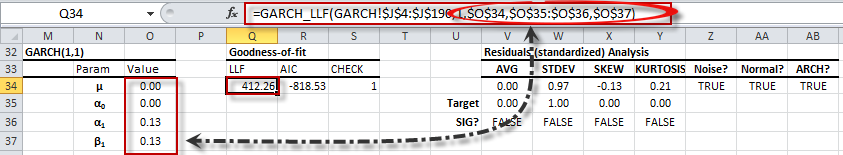In the right-most table (i.e. Residuals Diagnosis), the wizard created a series of statistical tests (formulas) for the standardized residuals (i.e. $\{\epsilon_t\}$ ) to help us verify the GARCH assumption:

$$\epsilon_t\sim\textrm{i.i.d}\sim\Phi(0,1)$$

The generated formulas reference the model’s parameters cells and input data cells range, so when you calibrate (or modify) the values of the model’s parameters, the statistical test results reflect the model parameters’ latest values.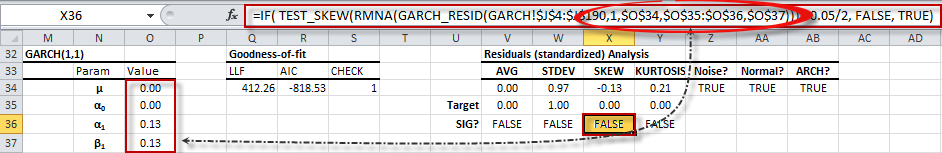## What's Next?

A quick recap: we’ve analyzed the input data statistical properties and come up with a suspect model: GARCH (1, 1). Now, we need to answer the following questions:

1. What are the optimal values for GARCH(1,1), given the input data?
2. Given the calibrated model, how well does the model fit the input data? Do the residuals address the assumption(s) of the underlying model?
3. Are there similar models to consider (e.g. EGARCH, GARCH-M, etc.)? How do we rank and ultimately decide which of them to use?

As you may have guessed, our analysis has reached a new phase: the model identification phase. For now, let’s address the first two questions:

• In module six (6), we will address the calibration process
• In module seven (7), we will visit the residuals diagnosis in greater detail and validate the model’s assumptions.